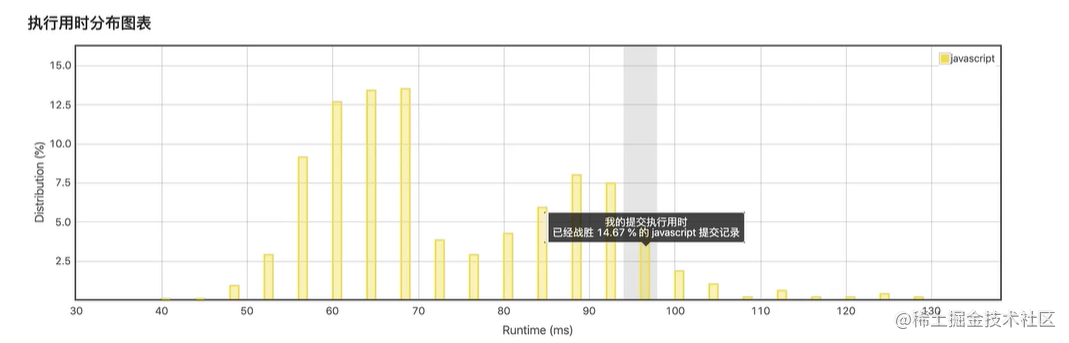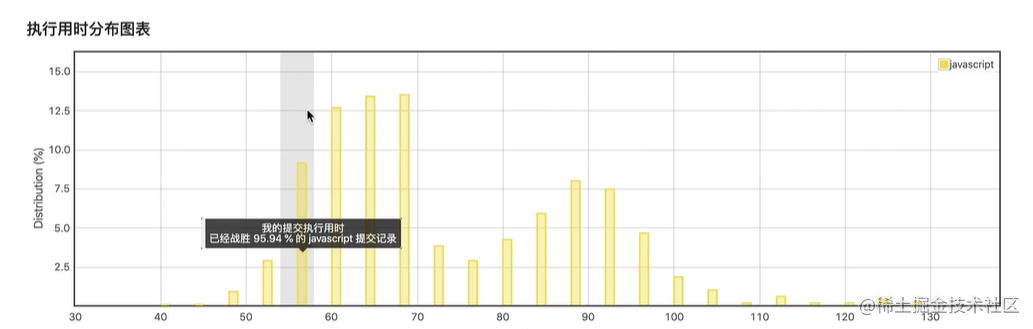# 算法-斐波那契数列

## 二、递归

``````var fib = function(N) {
if(N == 1 || N == 0) {
return N;
}
return fib(N-1) + fib(N-2);//递推公式
}• 当给你的N等于1或者等于0的时候直接输出
• 否则就用递归的方式拿到N前一个数字和N前两个数字，然后计算出这两个数字相加之和就是当前的N。

## 三、递推

• 顺推：根据已知的条件，求出需要解决的结果。
• 逆推：根据目前的问题，逐步求出条件。

``````var fib = function(N) {
let cache = [];
for(let i = 0;i <= N;i++) {
if(i == 1 || i == 0) {
cache[i] = i;
}else {
cache[i] = cache[i-1]+cache[i-2]
}
}
return cache[N]
}